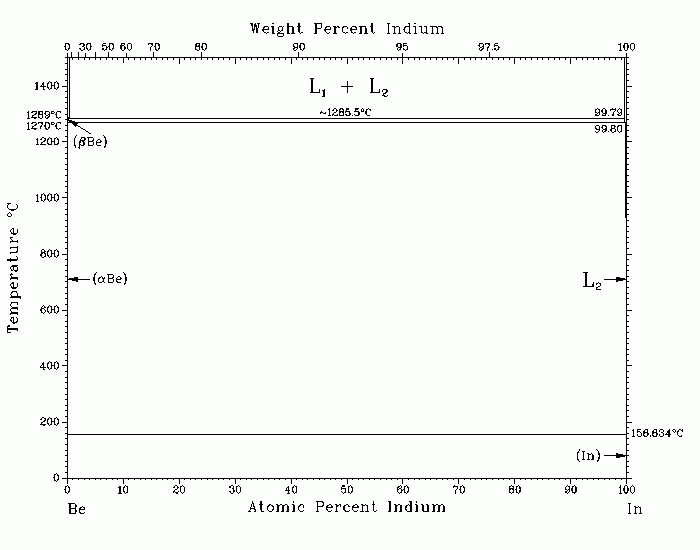# Фазовая диаграмма системы Be-InBe-In (Beryllium-Indium) H. Okamoto and L.E. Tanner The assessed Be-In phase diagram is based on [52Ell]. There is a very wide miscibility gap within the liquid range. The monotectic temperature is believed to be very close to the melting point of Be. Due to the wide scatter in observed arrest temperatures, [52Ell] suggested that the monotectic temperature is very close to the melting point of Be. The assessed diagram is a tentative phase diagram calculated on the basis of a regular solution model. No solubility is assumed for the terminal phases. According to this model, the monotectic point is calculated as 0.2 at.% In and 1285.5 C. The calculated maximum temperature of the liquid miscibility gap is 4538 C, significantly higher than the boiling point of either Be (2472 C) or In (2073 C). The melting point of bBe and the bBe = aBe allotropic transformation temperature are 1289 с 5 and 1270 с 6 C, respectively. No solubility of In in Be was found in an alloy quenched from 1000 C [52Ell]. [52Ell] measured the solubility of Be in liquid In as 0.009 at.% Be at 540 C, 0.013 at.% at 580 and 800 C, 0.025 at.% at 1000 C, and 0.13 at.% at 1200 C. The model indicates that the monotectic point is very close to the Be-rich end of the system. The three arrest temperatures of a 0.86 at.% In alloy, observed by [52Ell] during cooling, may correspond to (1) the liquidus, (2) the L1 = L2 + (bBe) monotectic, and (3) the (bBe) = L + (aBe) catatectic temperatures, respectively. 52Ell: R.O. Elliott and E.M. Cramer, U.S. At. Energy Comm. AECU-3022, 10 p ( 1952). Published in Phase Diagrams of Binary Beryllium Alloys, 1987. Complete evaluation contains 1 figure, 3 tables, and 2 references. 1